# 20220818151924_PPT01 - The Logic of Compound and Quantitative Statement.pptx

2 de Jun de 2023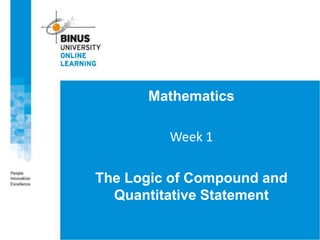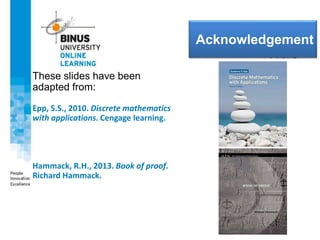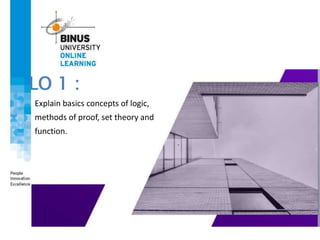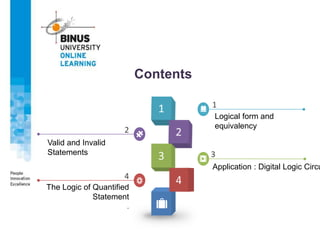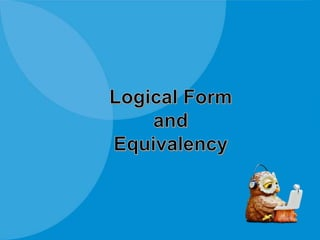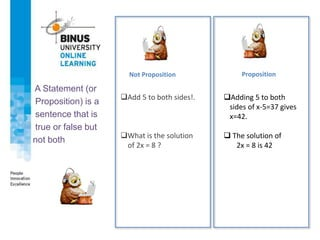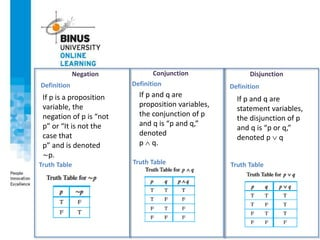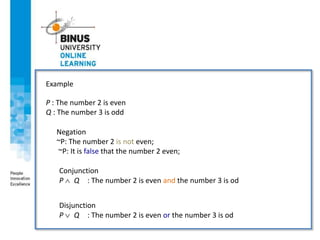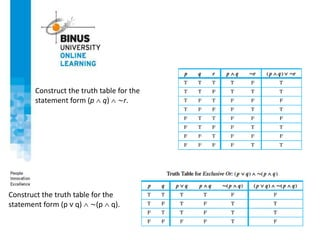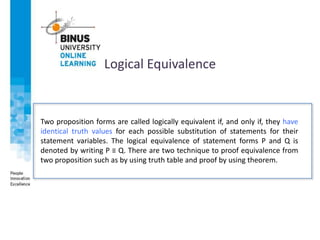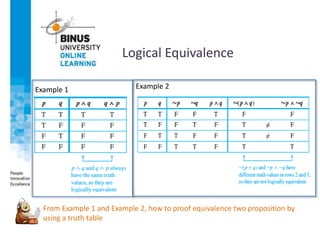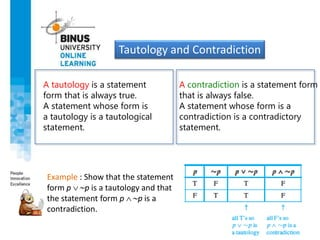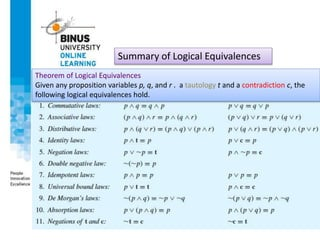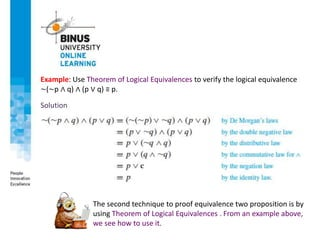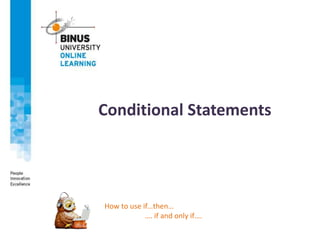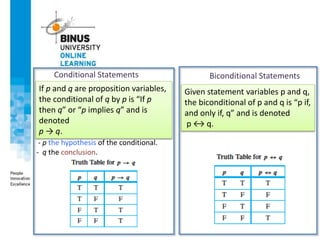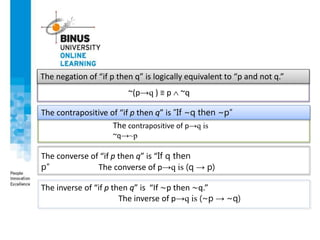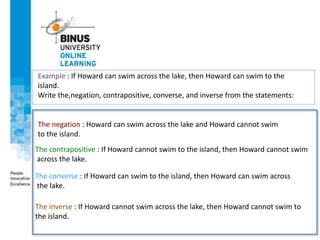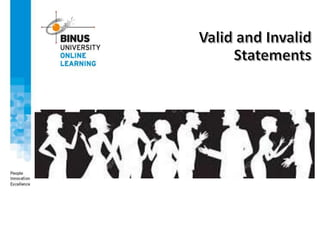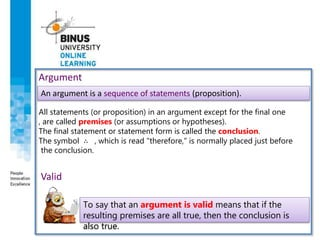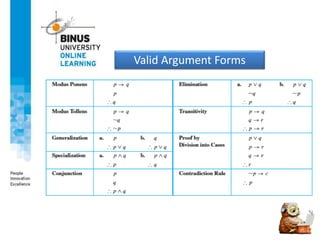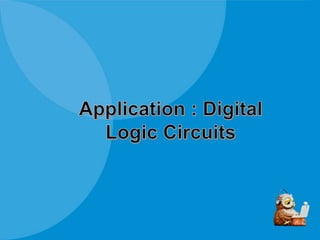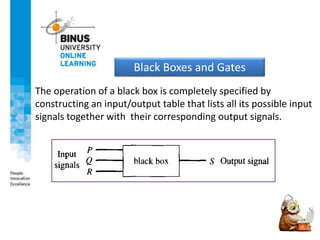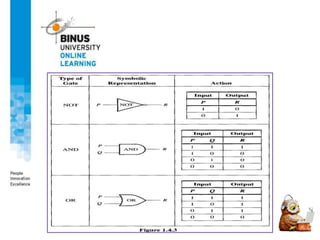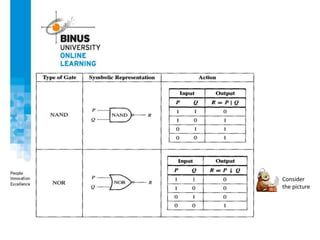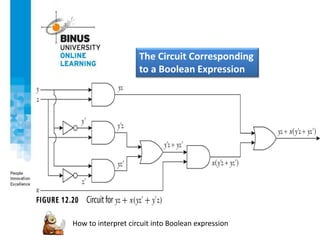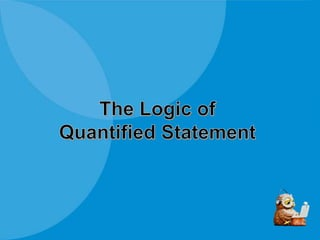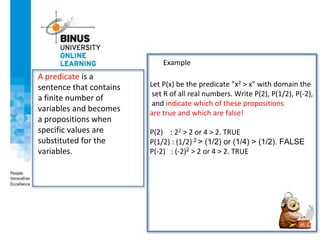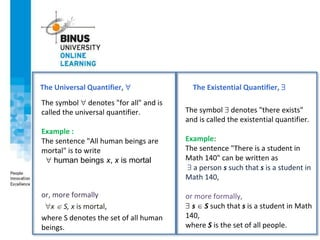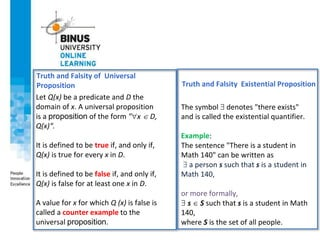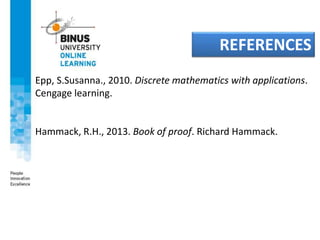1 de 33

### 20220818151924_PPT01 - The Logic of Compound and Quantitative Statement.pptx

• 1. Mathematics Week 1 The Logic of Compound and Quantitative Statement
• 2. <<Title>> Acknowledgement These slides have been adapted from: Epp, S.S., 2010. Discrete mathematics with applications. Cengage learning. Hammack, R.H., 2013. Book of proof. Richard Hammack.
• 3. LO 1 : Explain basics concepts of logic, methods of proof, set theory and function. .
• 4. Contents Logical form and equivalency 1 2 3 The Logic of Quantified Statement . 4 Application : Digital Logic Circu Valid and Invalid Statements
• 6. LOGIC Logic is a systematic way of thinking that allow us to deduce new information from old information and to parse the meaning of sentences
• 7. Not Proposition Proposition Add 5 to both sides!. What is the solution of 2x = 8 ? Adding 5 to both sides of x-5=37 gives x=42.  The solution of 2x = 8 is 42 A Statement (or Proposition) is a sentence that is true or false but not both
• 8. Negation Conjunction Disjunction Definition Truth Table Definition Definition If p is a proposition variable, the negation of p is “not p” or “It is not the case that p” and is denoted ∼p. If p and q are proposition variables, the conjunction of p and q is “p and q,” denoted p  q. Truth Table Truth Table If p and q are statement variables, the disjunction of p and q is “p or q,” denoted p  q
• 9. Example P : The number 2 is even Q : The number 3 is odd Negation ~P: The number 2 is not even; ~P: It is false that the number 2 even; Conjunction P  Q : The number 2 is even and the number 3 is od Disjunction P  Q : The number 2 is even or the number 3 is od
• 10. Construct the truth table for the statement form (p  q)  ∼r. Construct the truth table for the statement form (p v q)  ∼(p  q).
• 11. Two proposition forms are called logically equivalent if, and only if, they have identical truth values for each possible substitution of statements for their statement variables. The logical equivalence of statement forms P and Q is denoted by writing P ≡ Q. There are two technique to proof equivalence from two proposition such as by using truth table and proof by using theorem. Logical Equivalence
• 12. Logical Equivalence Example 1 Example 2 From Example 1 and Example 2, how to proof equivalence two proposition by using a truth table
• 13. A tautology is a statement form that is always true. A statement whose form is a tautology is a tautological statement. Tautology and Contradiction A contradiction is a statement form that is always false. A statement whose form is a contradiction is a contradictory statement. Example : Show that the statement form p ∼p is a tautology and that the statement form p ∼p is a contradiction.
• 14. Theorem of Logical Equivalences Given any proposition variables p, q, and r . a tautology t and a contradiction c, the following logical equivalences hold. Summary of Logical Equivalences
• 15. Example: Use Theorem of Logical Equivalences to verify the logical equivalence ∼(∼p ∧ q) ∧ (p ∨ q) ≡ p. Solution The second technique to proof equivalence two proposition is by using Theorem of Logical Equivalences . From an example above, we see how to use it.
• 16. Conditional Statements How to use if…then… …. if and only if….
• 17. Conditional Statements Biconditional Statements If p and q are proposition variables, the conditional of q by p is “If p then q” or “p implies q” and is denoted p → q. - p the hypothesis of the conditional. - q the conclusion. Given statement variables p and q, the biconditional of p and q is “p if, and only if, q” and is denoted p ↔ q.
• 18. The negation of “if p then q” is logically equivalent to “p and not q.” The contrapositive of “if p then q” is “If ~q then ~p” The converse of “if p then q” is “If q then p“ The inverse of “if p then q” is “If ∼p then ∼q.” ~(p→q ) ≡ p  ~q The contrapositive of p→q is ~q→~p The converse of p→q is (q → p) The inverse of p→q is (~p → ~q)
• 19. The negation : Howard can swim across the lake and Howard cannot swim to the island. The contrapositive : If Howard cannot swim to the island, then Howard cannot swim across the lake. Example : If Howard can swim across the lake, then Howard can swim to the island. Write the,negation, contrapositive, converse, and inverse from the statements: The converse : If Howard can swim to the island, then Howard can swim across the lake. The inverse : If Howard cannot swim across the lake, then Howard cannot swim to the island.
• 21. An argument is a sequence of statements (proposition). Argument Valid To say that an argument is valid means that if the resulting premises are all true, then the conclusion is also true. All statements (or proposition) in an argument except for the final one , are called premises (or assumptions or hypotheses). The final statement or statement form is called the conclusion. The symbol ∴ , which is read "therefore," is normally placed just before the conclusion.
• 24. The operation of a black box is completely specified by constructing an input/output table that lists all its possible input signals together with their corresponding output signals. Black Boxes and Gates
• 27. The Circuit Corresponding to a Boolean Expression How to interpret circuit into Boolean expression
• 29. A predicate is a sentence that contains a finite number of variables and becomes a propositions when specific values are substituted for the variables. Let P(x) be the predicate "x2 > x" with domain the set R of all real numbers. Write P(2), P(1/2), P(-2), and indicate which of these propositions are true and which are false! P(2) : 22 > 2 or 4 > 2. TRUE P(1/2) : (1/2) 2 > (1/2) or (1/4) > (1/2). FALSE P(-2) : (-2)2 > 2 or 4 > 2. TRUE Example
• 30. The Universal Quantifier,  The symbol  denotes "for all" and is called the universal quantifier. Example : The sentence "All human beings are mortal" is to write  human beings x, x is mortal or, more formally x  S, x is mortal, where S denotes the set of all human beings. The Existential Quantifier,  The symbol  denotes "there exists" and is called the existential quantifier. Example: The sentence "There is a student in Math 140" can be written as  a person s such that s is a student in Math 140, or more formally,  s  S such that s is a student in Math 140, where S is the set of all people.
• 31. Truth and Falsity of Universal Proposition Let Q(x) be a predicate and D the domain of x. A universal proposition is a proposition of the form "x  D, Q(x)“. It is defined to be true if, and only if, Q(x) is true for every x in D. It is defined to be false if, and only if, Q(x) is false for at least one x in D. A value for x for which Q (x) is false is called a counter example to the universal proposition. Truth and Falsity Existential Proposition The symbol  denotes "there exists" and is called the existential quantifier. Example: The sentence "There is a student in Math 140" can be written as  a person s such that s is a student in Math 140, or more formally,  s  S such that s is a student in Math 140, where S is the set of all people.
• 32. Epp, S.Susanna., 2010. Discrete mathematics with applications. Cengage learning. Hammack, R.H., 2013. Book of proof. Richard Hammack. REFERENCES